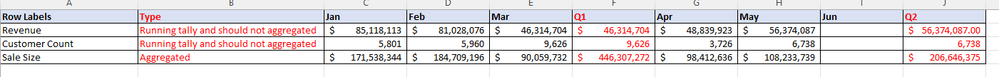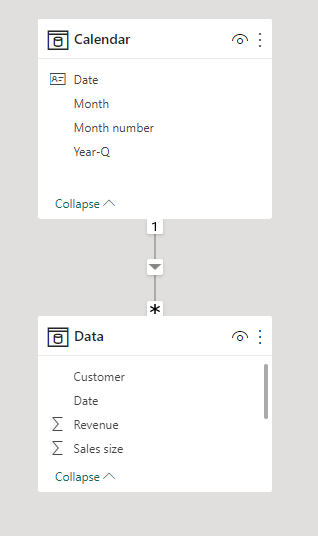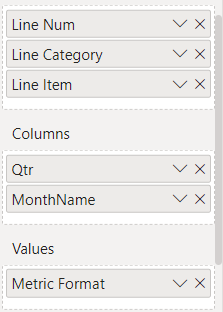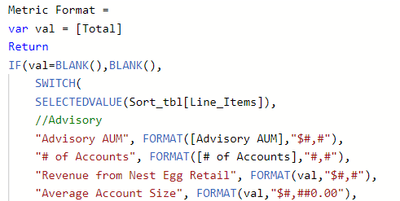cancel
Showing results for
Did you mean:Helper I

## Tally for my qtr totals

Hi Team ,

I have hit a wall and I need some help.

I need to create a measure that captures my quarterly totals but some are aggregated and some are not and are just based on the last entered value for that month. The aggregation is fine but when I don't know how to get the last value to show in the qtr total as I have displayed below.

I have a date dimension table connected to the sales table in my model.any help is greatly appreciated.

1 ACCEPTED SOLUTIONSuper User

Hi,

I am not sure if I understood your question correctly, but I tried to create a sample pbix file like below.

Please check the below picture and the attached pbix file.

I hope the below can provide some ideas on how to create a solution for your  datamodel.``````Revenue: =
SWITCH (
TRUE (),
ISINSCOPE ( 'Calendar'[Month number] ), SUM ( Data[Revenue] ),
ISINSCOPE ( 'Calendar'[Year-Q] ),
VAR _yearquarter =
MAX ( 'Calendar'[Year-Q] )
VAR _quartertable =
FILTER ( ALL ( 'Calendar' ), 'Calendar'[Year-Q] = _yearquarter )
VAR _lastnonblankmonth =
MAXX (
FILTER (
ADDCOLUMNS ( _quartertable, "@rev", CALCULATE ( SUM ( Data[Revenue] ) ) ),
[@rev] <> BLANK ()
),
'Calendar'[Month number]
)
RETURN
CALCULATE (
SUM ( Data[Revenue] ),
'Calendar'[Year-Q] = _yearquarter,
'Calendar'[Month number] = _lastnonblankmonth
)
)``````

``````Customoer count: =
SWITCH (
TRUE (),
ISINSCOPE ( 'Calendar'[Month number] ), DISTINCTCOUNT(Data[Customer]),
ISINSCOPE ( 'Calendar'[Year-Q] ),
VAR _yearquarter =
MAX ( 'Calendar'[Year-Q] )
VAR _quartertable =
FILTER ( ALL ( 'Calendar' ), 'Calendar'[Year-Q] = _yearquarter )
VAR _lastnonblankmonth =
MAXX (
FILTER (
ADDCOLUMNS ( _quartertable, "@count", CALCULATE ( DISTINCTCOUNT(Data[Customer]) ) ),
[@count] <> BLANK ()
),
'Calendar'[Month number]
)
RETURN
CALCULATE (
DISTINCTCOUNT(Data[Customer]),
'Calendar'[Year-Q] = _yearquarter,
'Calendar'[Month number] = _lastnonblankmonth
)
)``````

``````Sales size: =
SUM( Data[Sales size] )``````

If this post helps, then please consider accepting it as the solution to help other members find it faster, and give a big thumbs up.

3 REPLIES 3Helper I

Hi @Jihwan_Kim quick question. I noticed in my Matrix visual that when I drill up to the quarter level the values do not show. For the value I am also using a switch function for the values See the below example. I need to be able to drill up and show the data at the quarter level.Helper I

@Jihwan_Kim  to the rescue again you nailed it. thanks so much.Super User

Hi,

I am not sure if I understood your question correctly, but I tried to create a sample pbix file like below.

Please check the below picture and the attached pbix file.

I hope the below can provide some ideas on how to create a solution for your  datamodel.``````Revenue: =
SWITCH (
TRUE (),
ISINSCOPE ( 'Calendar'[Month number] ), SUM ( Data[Revenue] ),
ISINSCOPE ( 'Calendar'[Year-Q] ),
VAR _yearquarter =
MAX ( 'Calendar'[Year-Q] )
VAR _quartertable =
FILTER ( ALL ( 'Calendar' ), 'Calendar'[Year-Q] = _yearquarter )
VAR _lastnonblankmonth =
MAXX (
FILTER (
ADDCOLUMNS ( _quartertable, "@rev", CALCULATE ( SUM ( Data[Revenue] ) ) ),
[@rev] <> BLANK ()
),
'Calendar'[Month number]
)
RETURN
CALCULATE (
SUM ( Data[Revenue] ),
'Calendar'[Year-Q] = _yearquarter,
'Calendar'[Month number] = _lastnonblankmonth
)
)``````

``````Customoer count: =
SWITCH (
TRUE (),
ISINSCOPE ( 'Calendar'[Month number] ), DISTINCTCOUNT(Data[Customer]),
ISINSCOPE ( 'Calendar'[Year-Q] ),
VAR _yearquarter =
MAX ( 'Calendar'[Year-Q] )
VAR _quartertable =
FILTER ( ALL ( 'Calendar' ), 'Calendar'[Year-Q] = _yearquarter )
VAR _lastnonblankmonth =
MAXX (
FILTER (
ADDCOLUMNS ( _quartertable, "@count", CALCULATE ( DISTINCTCOUNT(Data[Customer]) ) ),
[@count] <> BLANK ()
),
'Calendar'[Month number]
)
RETURN
CALCULATE (
DISTINCTCOUNT(Data[Customer]),
'Calendar'[Year-Q] = _yearquarter,
'Calendar'[Month number] = _lastnonblankmonth
)
)``````

``````Sales size: =
SUM( Data[Sales size] )``````

If this post helps, then please consider accepting it as the solution to help other members find it faster, and give a big thumbs up.